# 勾股定理2000 多年前有一个关于三角形的神奇发现：

。。。。。。而每一边都画一个正方形，。。。。。。

。。。。。。最大的正方形的 面积正好是 其他两个正方形的面积的和！a2 + b2 = c2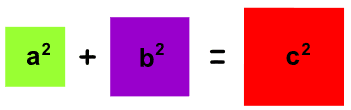• c三角形 最长的边
• ab其他两边

## 真的吗？

### 例子： 一个 "3，4，5" 三角形 有个直角。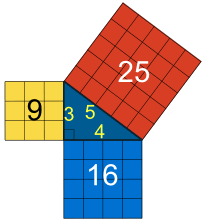我们来看看面积是不是一样的： 32 + 42 = 52 计算后是： 9 + 16 = 25 真的！多奇妙！## 怎样应用？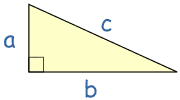a2 + b2 = c2

### 例子：解这个三角形。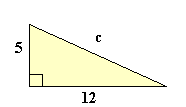a2 + b2 = c2 52 + 122 = c2 25 + 144 = c2 169 = c2 c2 = 169 c = √169 c = 13

### 例子：解这个三角形。a2 + b2 = c2 92 + b2 = 152 81 + b2 = 225 两边减 81： b2 = 144 b = √144 b = 12

### 例子：一个边长是 1 的正方形的 对角线距离 是什么？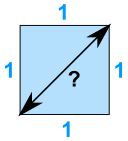a2 + b2 = c2 12 + 12 = c2 1 + 1 = c2 2 = c2 c2 = 2 c = √2 = 1.4142...

### 例子：这个三角形有直角吗？这对吗？ a2 + b2 = c2 a2 + b2 = 102 + 242 = 100 + 576 = 676 c2 = 262 = 676 它们相等，所以。。。。。。 对，这个三角形有直角！

### 例子： 一个 8, 15, 16 的三角形有直角吗？

82 + 152 = 162 吗？

• 82 + 152 = 64 + 225 = 289,
• 但 162 = 256

### 例子：这个三角形有直角吗？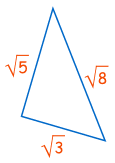这对吗？ a2 + b2 = c2 (√3)2 + (√5)2 = (√8)2 吗？ 3 + 5 = 8 吗？ 当然！ 这个 是 直角三角形

## 你可以自己证明这个定理！

• 在纸上画一个直角三角形，周围多留些空间。
• 在斜边（最长的一边）上画一个正方形
• 在斜边的另一边画同样大小的正方形
• 按动画的指示画线，像这样：
•• 剪下图形
• 把图形拼起来，看看可不可以证明大的正方形的面积是另外两个正方形的总和

## 另外一个非常简单的证明变成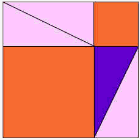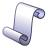历史附注： 在西方，这个定理叫毕达哥拉斯定理，但印度、希腊、中国和巴比伦的数学家都在毕达哥拉斯在世以前很久已熟悉这个定理！